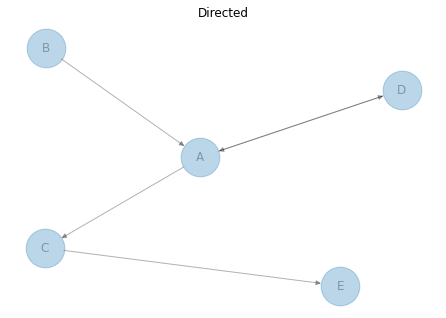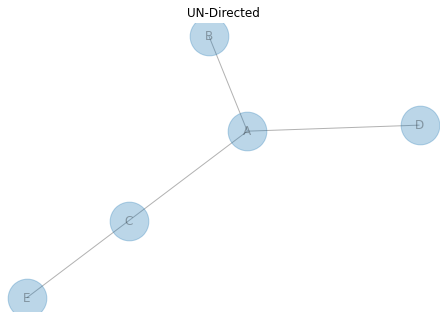Network charts can be split into 2 main categories: directed and undirected networks.

If it is directed, there is a notion of flow between 2 nodes, thus leaving a place to go somewhere else. Like money goes from company A to company B. That’s why you can see (kind of) arrows on the left chart, it gives the direction. The flow goes from B to A for example.

If it is undirected, there is just a link between 2 nodes, like mister A and mister B are friend.

When you build your graph, you have to use the function that suits your need: `Graph()` is used for undirected (default), `DiGraph()` is used for directed graph.

``````# libraries
import pandas as pd
import numpy as np
import networkx as nx
import matplotlib.pyplot as plt

# ------- DIRECTED

# Build a dataframe with your connections
# This time a pair can appear 2 times, in one side or in the other!
df = pd.DataFrame({ 'from':['D', 'A', 'B', 'C','A'], 'to':['A', 'D', 'A', 'E','C']})

# Build your graph. Note that we use the DiGraph function to create the graph!
G=nx.from_pandas_edgelist(df, 'from', 'to', create_using=nx.DiGraph() )

# Make the graph
nx.draw(G, with_labels=True, node_size=1500, alpha=0.3, arrows=True)
plt.title("Directed")
plt.show()

# ------- UNDIRECTED

# Build a dataframe with your connections
# This time a pair can appear 2 times, in one side or in the other!
df = pd.DataFrame({ 'from':['D', 'A', 'B', 'C','A'], 'to':['A', 'D', 'A', 'E','C']})

# Build your graph. Note that we use the Graph function to create the graph!
G=nx.from_pandas_edgelist(df, 'from', 'to', create_using=nx.Graph() )

# Make the graph
nx.draw(G, with_labels=True, node_size=1500, alpha=0.3, arrows=True)
plt.title("UN-Directed")
plt.show()``````## Contact & Edit

👋 This document is a work by Yan Holtz. You can contribute on github, send me a feedback on twitter or subscribe to the newsletter to know when new examples are published! 🔥